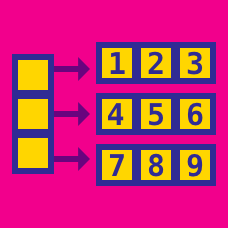Computer Science

# Linear Data Structures

#### Challenge Quizzes

Doubly linked lists are useful for more than just inserting and removing elements from the head and tail of the list. They can maintain a list of elements that allows for insertion and removal to the interior of the list

Given a node $v$ of a doubly linked list (which is currently followed by $w$), we want to insert a new node $z$ immediately after $v$. Concretely, we want to write a function insert(v) which takes a node, $v$, and inserts a new node after $v$. The result should be a list where $v$'s next points to $z$, $z$'s next points to $w$, $w$'s prev points to $z$, and $z$'s prev points to $v$.

If we execute these events in the wrong order, we can erase crucial information that will break the list. How should the following steps be ordered so that they correctly insert a node in-the middle of a doubly linked list?

• A Make $z$'s prev point to $v$.
• B Make $v$'s next point to $z$.
• C Make $w$'s prev point to $z$.
• D Make $z$'s next point to $w$.

Consider a circularly linked list. Which of the following methods below removes the node after the cursor? The cursor is a special node which allows us to have a place to start from if we ever need to traverse a circularly linked list.

  1 2 3 4 5 6 7 8 9 10 11 node A(node N){ Node oldNode = cursor.getNext(); if (oldNode == cursor) cursor = node.getNext() else{ cursor.setPrev(oldNode.getNext()); oldNode.setNext(null); } size --; return oldNode; } 

 1 2 3 4 5 node B(Node N){ Node oldNode = N oldNode.setNext(null) return oldNode; } 

  1 2 3 4 5 6 7 8 9 10 11 node C(Node N){ Node oldNode = cursor.getNext(); if (oldNode == cursor) cursor = null else{ cursor.setNext(oldNode.getNext()); oldNode.setNext(null); } size --; return oldNode; } 

  1 2 3 4 5 6 7 8 9 10 11 node D(Node N){ Node oldNode = N; if (oldNode == cursor) cursor = null else{ cursor.setNext(N); oldNode.setNext(null); } size --; return oldNode; } 

Given a singly linked list, write a program the shifts every node $k$ unit to the left.

For example

When 4$\rightarrow$6$\rightarrow$8$\rightarrow$9 is shifted $2$ units left it becomes 8$\rightarrow$9$\rightarrow$4$\rightarrow$6

What will the following linked list look like when each node is shifted $4432897427834$ to the left.

34$\rightarrow$17$\rightarrow$17$\rightarrow$74$\rightarrow$83$\rightarrow$59$\rightarrow$39

×